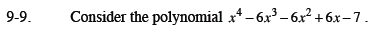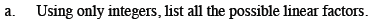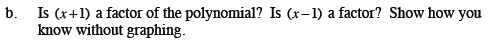Home > CCA2 > Chapter 9 > Lesson 9.1.1 > Problem9-9

9-9.
1. Consider the polynomial x4 − 6x3 − 6x2 + 6x − 7. Homework Help ✎

1. Using only integers, list all the possible linear factors.

2. Is (x +1) a factor of the polynomial? Is (x − 1) a factor? Show how you know without graphing.Use the Integral Zero Theorem found in the Math Notes box in Lesson 8.3.2. There are 4 answers!Long divide each of the factors into the polynomial. If you have no remainder, then it was a factor of the polynomial.